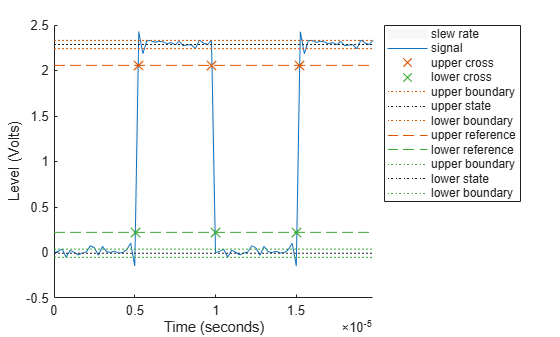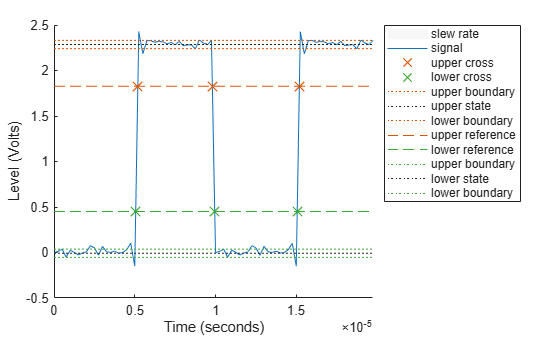# slewrate

Slew rate of bilevel waveform

## Syntax

S = slewrate(X) S = slewrate(X,Fs) S = slewrate(X,T) [S,LT,UT] = slewrate(...) [S,LT,UT,LL,UL] = slewrate(...) S = slewrate(...,Name,Value) slewrate(...) 

## Description

S = slewrate(X) returns the slew rate for all transitions found in the bilevel waveform, X. The slew rate is the slope of the line connecting the 10% and 90% reference levels. The sample instants of X are the indices of the vector. To determine the transitions, slewrate estimates the state levels of the input waveform by a histogram method. slewrate identifies all regions that cross the upper-state boundary of the low state and the lower-state boundary of the high state. The low-state and high-state boundaries are expressed as the state level plus or minus a multiple of the difference between the state levels. See State-Level Tolerances.

S = slewrate(X,Fs) specifies the sample rate, Fs, in hertz. The first time instant in X corresponds to t=0.

S = slewrate(X,T) specifies the sample instants in the vector, T. The length of T must equal the length of X.

[S,LT,UT] = slewrate(...) returns the time instants when the waveform crosses the lower-percent reference level, LT, and upper-percent reference level, UT. If you do not specify lower- and upper-percent reference levels, the levels default to 10% and 90%.

[S,LT,UT,LL,UL] = slewrate(...) returns the waveform values that correspond to the lower-reference levels, LL, and upper-reference levels, UL.

S = slewrate(...,Name,Value) returns the slew rate for all transitions with additional options specified by one or more Name,Value pair arguments.

slewrate(...) plots the bilevel waveform and darkens the regions of each transition where the slew rate is computed. The plot marks the lower- and upper-reference level crossings and associated reference levels. The plot indicates the state levels and associated lower and upper tolerances.

## Input Arguments

 X Bilevel waveform as a real-valued column or row vector. If the input waveform does not have at least one transition, slewrate returns an empty matrix. Fs Sample rate in hertz. T Vector of sample instants. The length of T must equal the length of the bilevel waveform, X.

### Name-Value Pair Arguments

 'PercentReferenceLevels' Percent reference levels. See Percent Reference Levels for a definition. Default: [10,90] 'StateLevels' Low- and high-state levels. StateLevels is a 1-by-2 real-valued vector. The first element is the low-state level. The second element is the high-state level. If you do not specify low- and high-state levels, slewrate estimates the state levels from the input waveform using the histogram method. 'Tolerance' Tolerance levels (lower and upper state boundaries) expressed as a percentage. See State-Level Tolerances. Default: 2

## Output Arguments

 S Slew rates as real-valued scalars. A positive slew rate indicates that the upper-percent reference level occurs later than the lower-percent reference level. A negative slew rate indicates that the upper-percent reference level occurs before the lower-percent reference level. LT Time instants when signal crosses the lower percent reference level. If you do not specify the lower percent reference levels with the 'PercentReferenceLevels' name-value pair, the lower percent reference level is 10%. UT Time instants when signal crosses the upper-percent reference level. If you do not specify the upper-percent reference levels with the 'PercentReferenceLevels' name-value pair, the upper-percent reference level is 90%. LL Waveform values at the lower-reference level. UL Waveform values at the upper-reference level.

## Examples

collapse all

Use slewrate with no output arguments to plot the slew rate information for a step waveform sampled at 4 MHz.

Load the transitionex.mat file and compute the slew rate. Annotate the slew rate in a plot of the waveform.

load('transitionex.mat','x','t') slewrate(x,t)ans = 1.0310e+07 

Create a bilevel waveform with three transitions, two positive and one negative. The sample rate is 4 MHz. Obtain the slew rates for the three transitions.

load('transitionex.mat','x') fs = 4e6; y = [x;fliplr(x)]; t = (0:length(y)-1)/4e6; S = slewrate(y,t)
S = 3×1 107 × 1.0310 -0.9320 1.0310 

Annotate the result on a plot of the waveform.

slewrate(y,t);Return the lower- and upper-transition times for a three-transition waveform sampled at 4 MHz.

load('transitionex.mat','x') fs = 4e6; y = [x;fliplr(x)]; t = (0:length(y)-1)/fs; [~,LT,UT] = slewrate(y,t)
LT = 3×1 10-4 × 0.0504 0.0998 0.1504 
UT = 3×1 10-4 × 0.0521 0.0978 0.1521 

Repeat using the sample rate instead of the time vector.

[~,LT,UT] = slewrate(y,fs)
LT = 3×1 10-4 × 0.0504 0.0998 0.1504 
UT = 3×1 10-4 × 0.0521 0.0978 0.1521 

Annotate the result on a plot of the waveform.

slewrate(y,fs);Return the waveform values corresponding to the lower- and upper-reference levels for a three-transition waveform sampled at 4 MHz. Compute these values for 10% and 90%, the default levels.

load('transitionex.mat','x') fs = 4e6; y = [x;fliplr(x)]; t = (0:length(y)-1)/fs; [~,~,~,LL,UL] = slewrate(y,t)
LL = 0.2212 
UL = 2.0564 

Repeat the calculation for 20% and 80%. Annotate the result on a plot of the waveform

slewrate(y,t,'PercentReferenceLevels',[20 80]);collapse all

### Percent Reference Levels

If S1 is the low state, S2 is the high state, and U is the upper-percent reference level. The waveform value corresponding to the upper-percent reference level is

${S}_{1}+\frac{U}{100}\left({S}_{2}-{S}_{1}\right).$

If L is the lower-percent reference level, the waveform value corresponding to the lower percent reference level is

${S}_{1}+\frac{L}{100}\left({S}_{2}-{S}_{1}\right).$

### Slew Rate

The slew rate is the slope of a line connecting the upper- and lower-percent reference levels. Let tL denote the time instant when the waveform crosses the lower reference level and tU denote the time instant when the waveform crosses the upper percent reference level. Using the definitions for the upper and lower percent reference levels given in Percent Reference Levels, the slew rate is

$\frac{{S}_{1}+\frac{U}{100}\left({S}_{2}-{S}_{1}\right)-\left\{{S}_{1}+\frac{L}{100}\left({S}_{2}-{S}_{1}\right)\right\}}{{t}_{U}-{t}_{L}}=\frac{U-L}{100}\frac{{S}_{2}-{S}_{1}}{{t}_{U}-{t}_{L}}.$

When tL occurs earlier than tU, the slew rate is positive. When tU occurs earlier than tL, the slew rate is negative.

### State-Level Tolerances

Each state level can have associated lower- and upper-state boundaries. These state boundaries are defined as the state level plus or minus a scalar multiple of the difference between the high state and the low state. To provide a useful tolerance region, the scalar is typically a small number such as 2/100 or 3/100. In general, theregion for the low state is defined aswhereis the low-state level andis the high-state level. Replace the first term in the equation withto obtain thetolerance region for the high state.

The following figure illustrates lower and upper 2% state boundaries (tolerance regions) for a positive-polarity bilevel waveform. The red dashed lines indicate the estimated state levels.## References

 IEEE® Standard on Transitions, Pulses, and Related Waveforms, IEEE Standard 181, 2003.